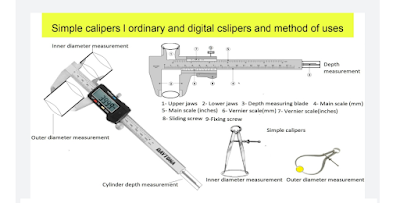## Thursday, September 7, 2023

### Simple calipers and vernier calipers, method of uses and calculations

Calipers:

The calipers are very useful instruments. These instruments are used to measure the diameter of the cylinder, bore, bearing size, and small distance accurately. There are two types of calipers used in the industry:

1- Simple calipers
2- Vernier calipers

Simple calipers:

These calipers are used to measure the inner and outer diameter of bigger size cylinders. Suppose that we have measured the outer diameter of a big size solid roller or cylinder and there are pivots on each side of the roller. This roller rotates on its pivots. In this case, we can not use a measuring scale for diameter measurement. The role of simple callipers comes into play now. You can measure the outer diameter of this roller accurately with the help of the simple callipers. See the below diagram:

There are two simple callipers used to measure the cylinder Or roller diameters. You can see clearly in the above figure.

The simple calipers consist of two legs bent at the bottom. The top ends of both leg are connected to each other with the help of a leaf springs. This leaf spring tends to keep both legs close to each other. A adjusting screw fitted near the top of the calipers helps to increase or decrease the gap between two legs as per requirement.

The calliper having legs bent inside the instrument is used for outer diameter measurement. The object ( cylinder or roller) is fixed between the legs ( jaws) and the screw is tightened gently. The bent end of each leg ( jaw) just touches the cylinder Or roller surface. The jaws should just touch the surface not put pressure on the surface. Now, the instrument is taken out and the distance between two jaws( legs) is measured with the help if measuring scale graduated in the millimeters.

The calipers having bottom ends of legs (jaws) bent outside direction of the instrument are used to measure the inner diameter of cylinder. The measuring procedure is same as stated for outer diameter measurements.
You can easily measure inner and outer diameter by following the given picture as above.

Vernier caliper:

This instrument is used to measure small dimensions accurately. We can measure inner diameter, outer diameter, and depth of cylinder accurately  by this vernier caliper. The structure of this instrument is given below:
1- It is constructed of two scales.
2- A fixed scale graduated inver millimeters is called main scale.
3- A sliding or moving scale graduated in certain no. Of divisions is called vernier scale.
4- The number of divisions in the vernier scale is more than one of the smallest divisions present in the main scale. If the vernier scale has 50 divisions then these 50 divisions of vernier scale will be equal to 49 divisions of main scale.
5- It had two upper jaws. These upper jaws are used to measure inner diameter of a cylinder.
6- It has two lower jaws. These lower jaws are used to measure outer diameter of a cylinder or a roller.
7-  A depth measuring blade is used to measure the  depth of a cylinder.
8- A sliding screw helps to move the vernier scale left to right or vice versa.
9- A fixing screw helps to fix the position of vernier scale during dimension measurements.

Measurement procedure:

1 - First of all see carefully the whole instrument.
2- See the least count of vernier calliper written on it.
3- If the least count is not mentioned, we calculate the same by formula.
4- Close the fixed and movable jaws of instrument.
5- Zero point of main scale gets matched with zero point of vernier scale. If there is found any error, this zero error is rectified first.
6- The upper line of the main scale is graduated in inches and lower line of main scale is graduated in millimeters.
7- Count the number of divisions marked on the vernier scale.
8- Fix the object between lower jaws if we are measuring outer diameter.
9- If you are measuring inner diameter of an object, fix the object between upper jaws.
10- Use depth measuring blade in case of depth measurement of a cylinder.
11- Finally, fix the fixing screw.

Observations:

No. of divisions in vernier scale = 50
Value of one division of vernier scale = 1 mn

Calculations:

Least count= (Value of one division of vernier scale) / (No. of divisions in vernier scale)
= 1/50
= 0.02 mn
Main scale reading = 25 mn

Vernier scale reading = 38 no. Division( it means the 38 number division is getting matched with any division of any one division of main scale)

Cylinder dia ( mn) = main scale reading + ( vernier scale reading x least count)
= 25 + ( 38 x 0.02)
= 25 + 0.76
Diameter= 25.76 m.m.

Digital vernier caliper:

This instrument measures dimension accurately. It gives direct reading of dimension of the object. A digital display is mounted on the vernier scale. Remaining procedure and structure are same as that of simple vernier caliper.